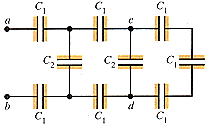# Capacitors in Series and Parallel homework

SuperCass

## Homework Statement

In Fig. 25-22, each capacitance C1 is 7.2 µF, and each capacitance C2 is 4.8 µF(a) Compute the equivalent capacitance of the network between points a and b.

(b) Compute the charge on each of the three capacitors nearest a and b when Vab = 450 V.

(c) With 450 V across a and b, compute Vcd.

Q=CV

## The Attempt at a Solution

I tried figuring the total capacitance, but I can't tell what is series and what is parallel! In series I know that the potential differences add up in series and they are the same in parallel, and the opposite is true for charge. Please help!